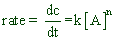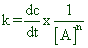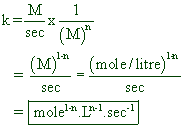# RATE LAW OR EXPRESSION; ORDER OF REACTION; MOLECULARITY

The differential equation that describes the mathematical dependance of rate of reaction on the concentration terms of the reactants is called rate law or rate expression or rate equation.

For a general reaction,

aA + bB + cC ----------> products

the rate law can be written as:

rate (r) = k[A]x[B]y[C]z

where

k= rate constant or specific rate

x, y and z are the orders with respect to A,B and C respectively.

## ORDER OF REACTION

Sum of the powers of the concentration terms in the rate equation is called overall order of the reaction

Hence the order of above reaction = x + y + z

* The order of a reaction and hence the rate law must be determined experimentally and cannot be predicted from the stoichiometric equation.

However in the cases of simple reactions, the rate expression can be written according to the stoichiometric equation. But the actual rate law must be proved experimentally.

* The order of reaction may have positive or negative or zero values.

The order with respect to an individual reactant may be some times fractional. However the order of the entire reaction is usually an integer.

* The rate law may or may not include concentration terms of all the reactants .

* Sometimes, the rate law may include the concentration terms of species like catalysts, which do not appear in their stoichiometric equations.

Depending on the order of reaction, the chemical reactions can be divided into zero, first, second & third order reactions as follows.

Zero order reactions: Total order of the reaction is zero.

Example:

1) Decomposition of NH3 on metal surfaces such as gold and molybdenum.r = k[NH3]0

or

r = k

First order reactions: Total order of the reaction is one.

Examples:

1) Thermal decomposition of Nitrogen pentoxide, N2O5

N2O5 --------> N2O4 + 1/2 O2

r = k[N2O5

2) Thermal decomposition of Sulfuryl chloride, SO2Cl2

SO2Cl2 ---------> SO2+Cl2

r = k [SO2Cl2

3) Thermal decomposition of Hydrogen peroxide, H2O2

H2O2 -----------> H2O + 1/2 O2

r = k[H2O2

Second order reactions: Total order of the reaction is two.

Examples:

1) Thermal decomposition of Nitrous oxide, N2O.

2N2O ---------> 2N2 + O2

r= k [N2O]2

2) Decomposition of Cl2O.

2Cl2O 2Cl2 + O

r= k [Cl2O]2

Third order reactions : Total order of the reaction is three.

Examples:

1) Reaction between NO and O2 to give NO2

2NO + O2 ---------> 2NO2

r = k[NO]2[O2

2) Reaction between NO and Cl2 to give NOCl

2NO + Cl2 ----------> 2NOCl

r = k[NO]2 [Cl2]

## RATE CONSTANT (k) & ITS UNITS

The rate of a reaction at the unit concentrations of all the reactants of the reaction is called specific rate (or) rate constant

i.e. rate = k,  when [reactants] = 1

Note: The rate constant of a reaction does not depend on the concentration and partial pressures of reactants. However it depends on the temperature. In general, increases with increase in the temperature.

Units of rate constant

For a general reaction,  A --------> products

Rate expression can be written as:where n = order of the reaction

OrTherefore units of k can be written as:i.e.,

For 1st order reaction   --------> sec-1

For 2nd order reaction  --------> L.mole-1sec-1

For 3rd order reaction   --------> L2.mole-2sec-1

For zero order reaction --------> mole .L-1sec-1

Note:

1) The unit for time can be expressed in minutes or hours or years also.

2) Also remember that 1 Litre = 1000 mL = 1000 cm3 = 1000 cc = 1 dm3

## MOLECULARITY

Elementary reactions: The chemical reactions which occur in one single step are called elementary reactions.

But most of the reactions occur in more than one step. The sequence of elementary steps that occur during a reaction is referred to as mechanism of that reaction.

Mechanism of a reaction: Consider a reaction, A ------> B , which is occurring in the following elementary steps:

i) A --------> X                 fast

ii) 2X -------> Y                slow (rate determining step)

iii) 2Y + A --------> 2B      fast

The above sequence of elementary steps is referred to as the mechanism of the reaction.

The slow step (ii) is called rate determining step.

Rate determining step: The elementary reaction with slowest rate is called rate determining step or rate limiting step. The rate of overall reaction depends on this step. It is also the step with highest activation energy.

Molecularity: The total number of atoms or molecules or ions taking part in an elementary reaction is called molecularity of that elementary reaction (or step).

E.g. The molecularities of elementary steps in the reaction mechanism proposed for the above reaction, A ------> B, are:

 Step Molecularity i) A --------> X 1 ii) 2X -------> Y 2 iii) 2Y + A --------> 2B 3

It is only possible to define the molecularity of an elementary step. But the molecularity of the overall reaction which involves more than one step cannot be defined.

However according to some authors, the molecularity of the rate determining step can be taken as the molecularity of the reaction in some cases.

* Molecularity is an integer.

* It cannot be zero or fractional.

* The maximum value observed is 3.

* It can be deduced theoretically from the proposed mechanism.

 Order Molecularity 1) Sum of the powers of concentration terms in rate expression is called order. 1) Total number of atoms or molecules or ions taking part in an elementary reaction is called molecularity 2) It may have zero or positive or negative or integer or fractional values. 2) It can only have integral values (1,2 or 3). Its value cannot be zero or fractional. 3) It is determined experimentally 3) It is deduced theoretically from the mechanism of the reaction.

 <  Factors affecting rate Chemical kinetics - TOC Integrated rate laws >